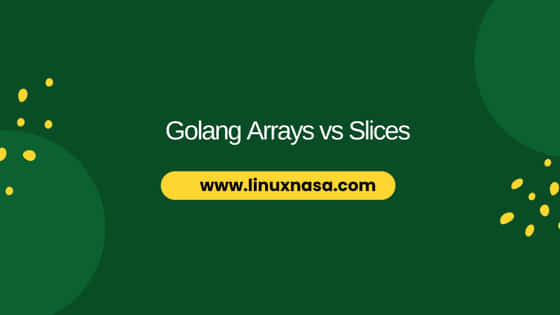# Golang Arrays vs Slices

In this tutorial, we will learn about Golang arrays vs slices. Bothe arrays and slices are data structures used for storing sequential elements. But there is subtle difference between the two data structure based on use cases and requirements. We will understand the difference between these two data structures based on many factors in the next section.## What is Array ?

In Golang, an array is a fundamental data structure that represents a fixed-size collection of elements of the same data type. Each element in an array is accessed by an index, which starts from 0 for the first element and increments by 1 for each subsequent elements. Arrays are used to store and manage a homogeneous sequence of values.

Due to array’s fixed size and pass by value nature, arrays are often replaced by another data structure in Golang, “slices” as slices offer more flexibility and memory efficiency.

## What is Slices ?

Also read: Slices in Golang: [14 Easy Examples]

Slice is a flexible and dynamic data structure that provides a more versatile way to work with sequences of elements compared to arrays. Slices are built on top of arrays and offer dynamic sizing, efficient memory usage and convenient operations. Now let’s look at some basic and key difference between array and slice data structure in Golang in the next section.

## Golang Arrays vs Slices

Also read: Go Run vs Go Build: [Explained with Example]

Now that we understand the basic definition of both data data structure `arrays` and `slices`, let us now look at some basic and key difference between two data structure. These differences will help you to choose the right data structure based on your use cases.

### Size

An Array has a fixed size determined at compile time and cannot be resized whereas Slice has a dynamic size and can be resized using `append` or by creating new slices.

```var arr int   //Array with fixed size
var slice []int    // Slice with dynamic size```

### Creation

Array is created using the syntax `[size]Type{values}` whereas Slice is created from an existing array or another slice using slicing or a slice literal.

```arr := int{4, 5, 6, 7, 8, 9}       //Creating an array
slice1 := arr[1:3]             // Creating a slice from an array
slice2 := []int{4, 5}       // Creating a slice using a slice literal```

### Passing to Functions

Array is passed by value which involves copying the entire array whereas Slice is passed by value but the value is a reference to the underlying array

```func modifyArray(arr int){
arr = 100
}

func modifySlice(slice []int){
slice = 100
}

arr := int{1, 2, 3}
modifyArray(arr)           //original array remains unchanged

slice := []int{1, 2, 3}
modifySlice(slice)          //original slice is modified```

### Appending Elements

The `append` function allows you to add elements to the end of a slice. This is a powerful feature that simplifies operations like building up data gradually without needing to pre-allocate memory.

```slice := []int{1, 2, 3}
slice = append(slice, 4, 5)         //Appending to a slice```

### Resizing

Array can not be resize once declared. A new array must be created if needed whereas Slice can be resized using `append` or slicing .

```slice := []int{1, 2, 3}
newSlice := slice[:2]              //Resizing a slice```

### Memory Efficiency

Array can be less memory-efficient if unused elements are allocated whereas Slice is more memory-efficient due to dynamic sizing and automatic resizing.

```arr := int{1, 2, 3}
slice := []int{1, 2, 3}```

### Iteration

Array iteration is done using indexes whereas Slices iteration is done either using indexes or the `range` keyword.

```arr := int{1, 2, 3}
for i :=0; i < len(arr); i++ {            //Iterating through an array
fmt.Println(arr[i])
}

slice := []int{4, 5, 6}
for index, value := range slice {
fmt.Printf("Index: %d, Value: %d\n", index, value)     //Iterating through slice using range
}```

### Nil Value

Array cannot be nil. It always contains elements whereas Slice can be nil, representing an empty slice with no underlying array.

`var emptySlice []int            //Nil slice`

### Multidimensional Structures

Array supports multidimensional arrays whereas Slice supports slices of slices, creating multidimensional structures.

```var matrix int                     //Multidimensional array
var matrixSlice [][]int                 //Multidimensional slice

```

### Copying

Array requires manual copying element by element whereas Slice can be copied using the `copy` function.

```source := []int{1, 2, 3}
destination := make([]int, len(source))
copy(destination, source)                   //destination now holds [1, 2, 3]```

## Summary

Please remember that arrays and slices have their own advantages and use cases, and the choice between them depends on the specific requirements of your program. using examples above, we demonstrated the practical differences between arrays  and slices in Go and illustrate the scenarios in which one might be more suitable than the other.

Categories GO